# Shape

A shape is an object that has some form of distinguishable external boundary, surface, or outline.

There are many ways to classify shapes; they can be classified as open or closed shapes; they can be classified by their dimensions, such as 2D or 3D.

## Closed shapes

The line segments or curves forming a closed shape are continuous, meaning there is no break. The lines/curves must also form a closed region to be considered a closed shape:

## Open shapes

The segments or curves of an open shape are not always connected. At least one endpoint of one side is not connected to the rest:

## 2D shapes

A 2D shape is a shape that lies in a plane and only has a length and a width, but no height or depth. 2D shapes are often classified as polygons and non-polygons.

### Polygon

A polygon is a closed plane figure formed by line segments. The following is a list of some polygons.

PolygonShape
Triangle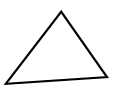Quadrilateral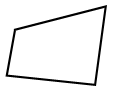Pentagon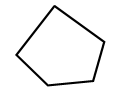Hexagon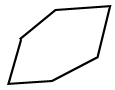This is not an exhaustive list of polygons. If there are n sides for a polygon, we call it an n-gon.

### Non-polygon

The following is a list of some non-polygon shapes.

NameShape
Angle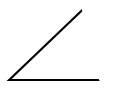Circle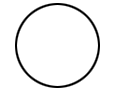Ellipse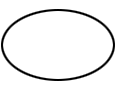Shapes with a curve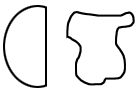Open 2D shapes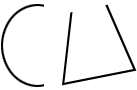Parabola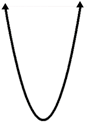## 3D shapes

The term "3D," or "three dimensional," is a term that is used to describe space figures. They occupy space and have volume if closed. 3D shapes can be classified as polyhedrons and non-polyhedrons.

### Polyhedron

A polyhedron is a closed solid whose faces are polygons. The following is one way to further classify polyhedra.

TypeExamples
Prisms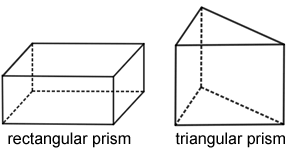Pyramids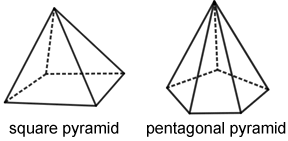Platonic solids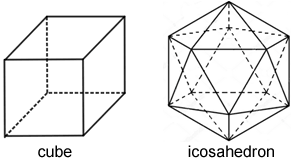There are many more prisms and pyramids, but only five platonic solids (regular, convex polyhedrons), the tetrahedron, cube, octahedron, dodecahedron, and icosahedron.

### Non-polyhedron

A non-polyhedron is a solid that has surfaces that are fully curved or partially curved.

TypeExamples
Partially curved surfaces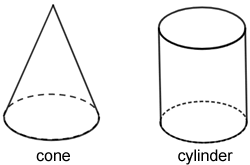Fully curved surfaces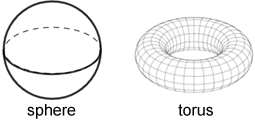Each shape above can be further classified. For example, a quadrilateral can be further classified as a parallelogram, rectangle, trapezoid, square, and more. Learn more about specific shapes and their classifications by navigating this website.